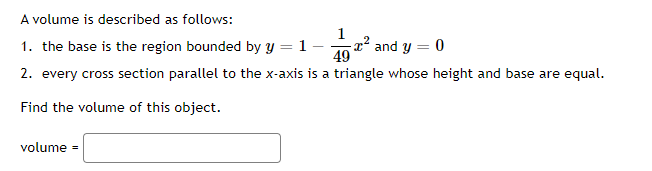### Create an Account

Home / Questions / A volume is described as follows: 1 1. the base is the region bounded by y = 1- tº and y =...

# A volume is described as follows: 1 1. the base is the region bounded by y = 1- tº and y = 0 49 2. every cross section parallel to the x-axis is a triangle whose height and base are equal. Find the vo

A volume is described as follows: 1 1. the base is the region bounded by y = 1- tº and y = 0 49 2. every cross section parallel to the x-axis is a triangle whose height and base are equal. Find the volume of this object. volume =May 16 2021 View more View LessSubscribe To Get Solution